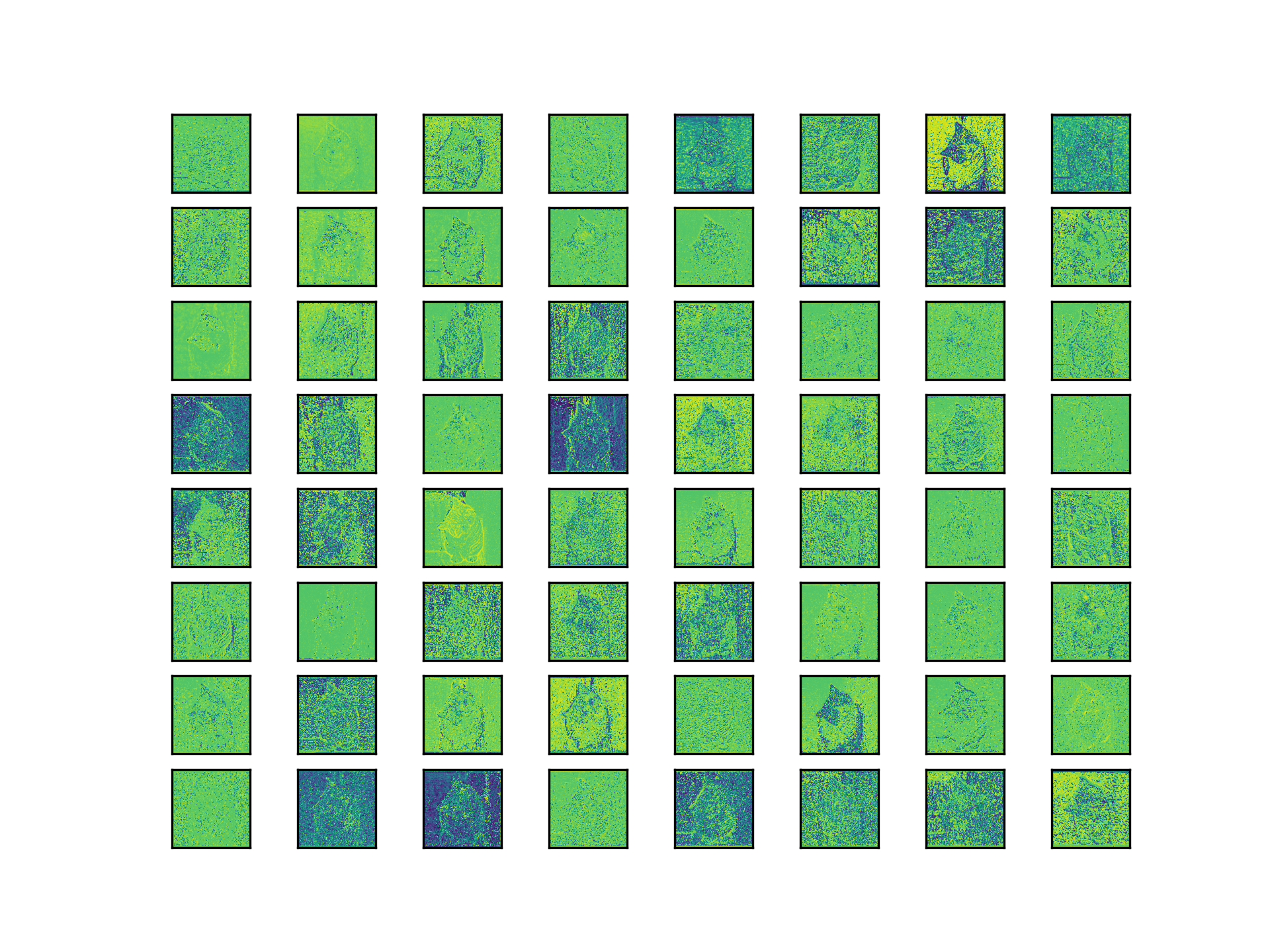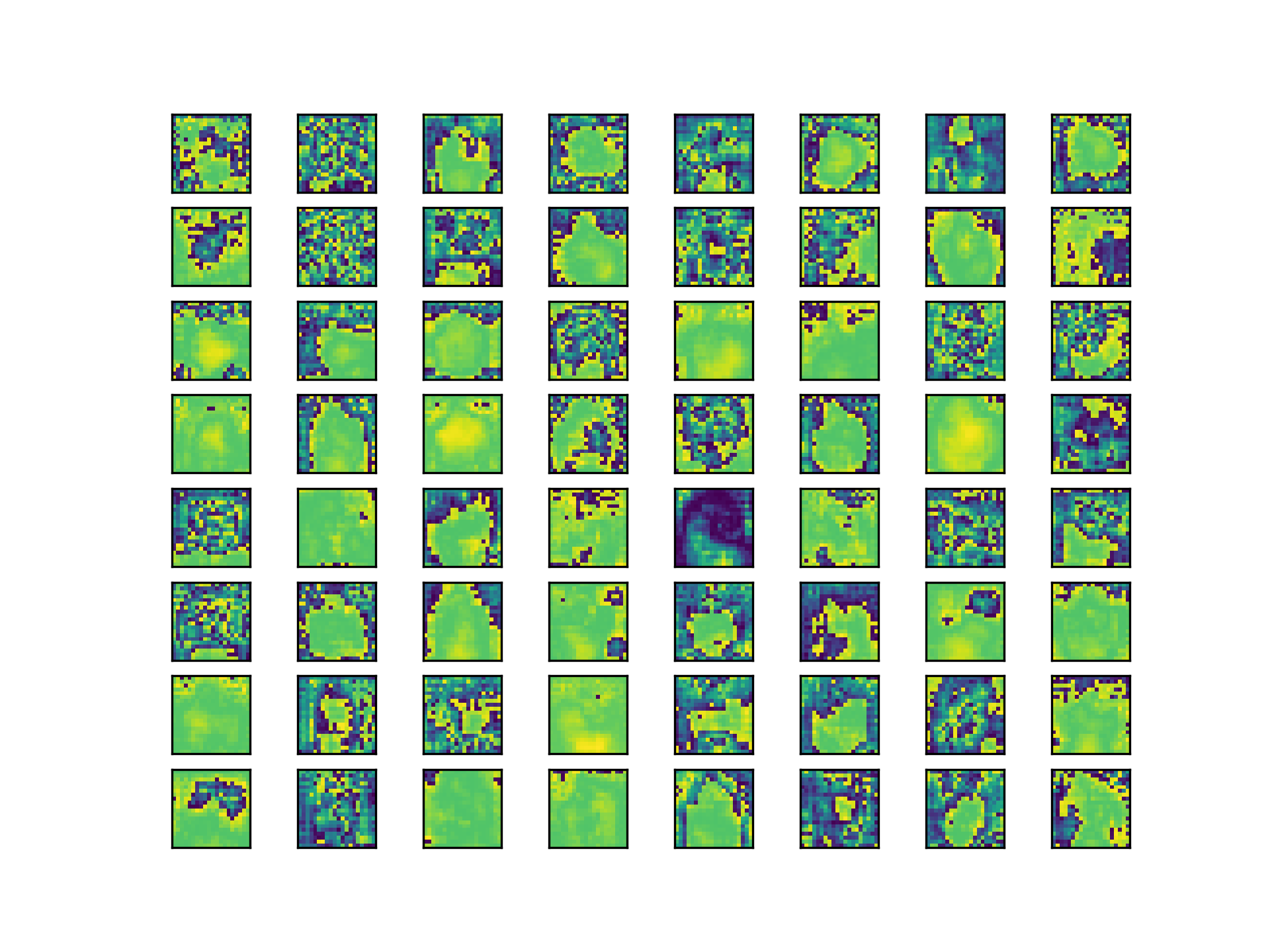1.使用方法

2.注意事项

# 一、效果图BTW, a single feature map may be in my opinion a shallow set of information, as you are looking at a 2d spatial slice but are not aptly observing relationships across the feature space (as the convolutions do).

I guess an analogy is that you would be viewing the R, G, B layers of a color image by themselves, when it helps to view them together to get the complete picture.

# 二、使用步骤

## 1.使用方法

使用方法很简单，只需要在utils中的general.py或者plots.py添加如下函数：

``````import matplotlib.pyplot as plt
from torchvision import transforms

def feature_visualization(features, model_type, model_id, feature_num=64):
"""
features: The feature map which you need to visualization
model_type: The type of feature map
model_id: The id of feature map
feature_num: The amount of visualization you need
"""
save_dir = "features/"
if not os.path.exists(save_dir):
os.makedirs(save_dir)

# print(features.shape)
# block by channel dimension
blocks = torch.chunk(features, features.shape, dim=1)

# # size of feature
# size = features.shape, features.shape

plt.figure()
for i in range(feature_num):
torch.squeeze(blocks[i])
feature = transforms.ToPILImage()(blocks[i].squeeze())
# print(feature)
ax = plt.subplot(int(math.sqrt(feature_num)), int(math.sqrt(feature_num)), i+1)
ax.set_xticks([])
ax.set_yticks([])

plt.imshow(feature)
# gray feature
# plt.imshow(feature, cmap='gray')

# plt.show()
plt.savefig(save_dir + '{}_{}_feature_map_{}.png'
.format(model_type.split('.'), model_id, feature_num), dpi=300)``````

`接着在models中的yolo.py中的这个地方：`

``````def forward_once(self, x, profile=False):
y, dt = [], []  # outputs
for m in self.model:
if m.f != -1:  # if not from previous layer
x = y[m.f] if isinstance(m.f, int) else [x if j == -1 else y[j] for j in m.f]  # from earlier layers

if profile:
o = thop.profile(m, inputs=(x,), verbose=False) / 1E9 * 2 if thop else 0  # FLOPS
t = time_synchronized()
for _ in range(10):
_ = m(x)
dt.append((time_synchronized() - t) * 100)
print('%10.1f%10.0f%10.1fms %-40s' % (o, m.np, dt[-1], m.type))

x = m(x)  # run
y.append(x if m.i in self.save else None)  # save output

if profile:
print('%.1fms total' % sum(dt))
return x``````

``````            feature_vis = True
if m.type == 'models.common.C3' and feature_vis:
print(m.type, m.i)
feature_visualization(x, m.type, m.i)``````

``model = attempt_load(weights, map_location=device)``

## 2.注意事项

``from utils.general import feature_visualization``

Model Summary: 476 layers, 87730285 parameters, 0 gradients

``model = Model(opt.cfg).to(device)``

``model = attempt_load(opt.weights, map_location=device)``

# 总结# 参考

pytorch特征图可视化

pytorch 提取卷积神经网络的特征图可视化

Pytorch中Tensor与各种图像格式的相互转化